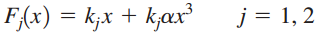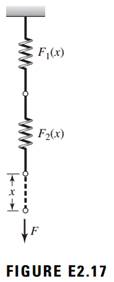### Create an Account

Home / Questions / Consider the two nonlinear springs in series shown in Figure E217 The force displacement r...

# Consider the two nonlinear springs in series shown in Figure E217 The force displacement relations for each spring are respectively a Obtain the expressions from which the equivalent spring

Consider the two nonlinear springs in series shown in Figure E2.17. The force-displacement relations for each spring are, respectively,a) Obtain the expressions from which the equivalent spring constant can be determined.

b) If F = 1000 N, k1 = 50,000 N/m, k2 = 25,000 N/m, and a = 2 m-2, determine the equivalent spring constant.Jun 25 2020 View more View LessSubscribe To Get Solution